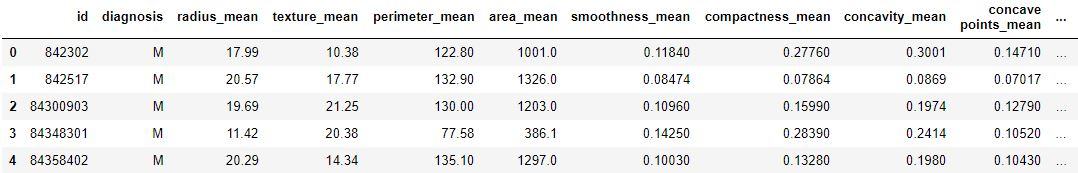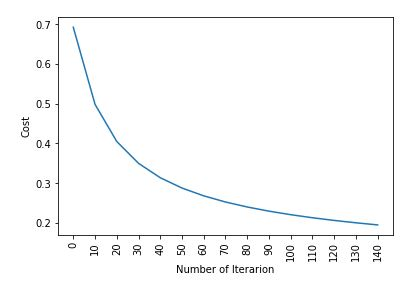Related Articles
ML | Kaggle Breast Cancer Wisconsin Diagnosis using Logistic Regression
• Last Updated : 07 Jun, 2019

Dataset :
It is given by Kaggle from UCI Machine Learning Repository, in one of its challenge
https://www.kaggle.com/uciml/breast-cancer-wisconsin-data. It is a dataset of Breast Cancer patients with Malignant and Benign tumor.

Logistic Regression is used to predict whether the given patient is having Malignant or Benign tumor based on the attributes in the given dataset.

Code : Loading Libraries
 `# performing linear algebra``import` `numpy as np `` ` `# data processing``import` `pandas as pd`` ` `# visualisation``import` `matplotlib.pyplot as plt`

Code : Loading dataset

 `data ``=` `pd.read_csv(``"..\\breast-cancer-wisconsin-data\\data.csv"``)`` ` `print` `(data.head)`

Output :Code : Loading dataset

 `data.info()`

Output :

```
RangeIndex: 569 entries, 0 to 568
Data columns (total 33 columns):
id                         569 non-null int64
diagnosis                  569 non-null object
radius_mean                569 non-null float64
texture_mean               569 non-null float64
perimeter_mean             569 non-null float64
area_mean                  569 non-null float64
smoothness_mean            569 non-null float64
compactness_mean           569 non-null float64
concavity_mean             569 non-null float64
concave points_mean        569 non-null float64
symmetry_mean              569 non-null float64
fractal_dimension_mean     569 non-null float64
radius_se                  569 non-null float64
texture_se                 569 non-null float64
perimeter_se               569 non-null float64
area_se                    569 non-null float64
smoothness_se              569 non-null float64
compactness_se             569 non-null float64
concavity_se               569 non-null float64
concave points_se          569 non-null float64
symmetry_se                569 non-null float64
fractal_dimension_se       569 non-null float64
radius_worst               569 non-null float64
texture_worst              569 non-null float64
perimeter_worst            569 non-null float64
area_worst                 569 non-null float64
smoothness_worst           569 non-null float64
compactness_worst          569 non-null float64
concavity_worst            569 non-null float64
concave points_worst       569 non-null float64
symmetry_worst             569 non-null float64
fractal_dimension_worst    569 non-null float64
Unnamed: 32                0 non-null float64
dtypes: float64(31), int64(1), object(1)
memory usage: 146.8+ KB
```

Code: We are dropping columns – ‘id’ and ‘Unnamed: 32’ as they have no role in prediction

 `data.drop([``'Unnamed: 32'``, ``'id'``], axis ``=` `1``)``data.diagnosis ``=` `[``1` `if` `each ``=``=` `"M"` `else` `0` `for` `each ``in` `data.diagnosis]`

Code : Input and Output data

 `y ``=` `data.diagnosis.values``x_data ``=` `data.drop([``'diagnosis'``], axis ``=` `1``)`

Code : Normalisation

 `x ``=` `(x_data ``-` `np.``min``(x_data))``/``(np.``max``(x_data) ``-` `np.``min``(x_data)).values`

Code : Splitting data for training and testing.

 `from` `sklearn.model_selection ``import` `train_test_split``x_train, x_test, y_train, y_test ``=` `train_test_split(``    ``x, y, test_size ``=` `0.15``, random_state ``=` `42``)`` ` `x_train ``=` `x_train.T``x_test ``=` `x_test.T``y_train ``=` `y_train.T``y_test ``=` `y_test.T`` ` `print``(``"x train: "``, x_train.shape)``print``(``"x test: "``, x_test.shape)``print``(``"y train: "``, y_train.shape)``print``(``"y test: "``, y_test.shape)`

Code : Weight and bias

 `def` `initialize_weights_and_bias(dimension):``    ``w ``=` `np.full((dimension, ``1``), ``0.01``)``    ``b ``=` `0.0``    ``return` `w, b`

Code : Sigmoid Function – calculating z value.

 `# z = np.dot(w.T, x_train)+b``def` `sigmoid(z):``    ``y_head ``=` `1``/``(``1` `+` `np.exp(``-``z))``    ``return` `y_head`

Code : Forward-Backward Propogation

 `def` `forward_backward_propagation(w, b, x_train, y_train):``    ``z ``=` `np.dot(w.T, x_train) ``+` `b``    ``y_head ``=` `sigmoid(z)``    ``loss ``=` `-` `y_train ``*` `np.log(y_head) ``-` `(``1` `-` `y_train) ``*` `np.log(``1` `-` `y_head)``    ``# x_train.shape  is for scaling``    ``cost ``=` `(np.``sum``(loss)) ``/` `x_train.shape[``1``]      `` ` `    ``# backward propagation``    ``derivative_weight ``=` `(np.dot(x_train, (``        ``(y_head ``-` `y_train).T))) ``/` `x_train.shape[``1``] ``    ``derivative_bias ``=` `np.``sum``(``        ``y_head``-``y_train) ``/` `x_train.shape[``1``]                 ``    ``gradients ``=` `{``"derivative_weight"``: derivative_weight,``                 ``"derivative_bias"``: derivative_bias}``    ``return` `cost, gradients`

Code : Updating Parameters

 `def` `update(w, b, x_train, y_train, learning_rate, number_of_iterarion):``    ``cost_list ``=` `[]``    ``cost_list2 ``=` `[]``    ``index ``=` `[]`` ` `    ``# updating(learning) parameters is number_of_iterarion times``    ``for` `i ``in` `range``(number_of_iterarion):``        ``# make forward and backward propagation and find cost and gradients``        ``cost, gradients ``=` `forward_backward_propagation(w, b, x_train, y_train)``        ``cost_list.append(cost)`` ` `        ``# lets update``        ``w ``=` `w ``-` `learning_rate ``*` `gradients[``"derivative_weight"``]``        ``b ``=` `b ``-` `learning_rate ``*` `gradients[``"derivative_bias"``]``        ``if` `i ``%` `10` `=``=` `0``:``            ``cost_list2.append(cost)``            ``index.append(i)``            ``print` `(``"Cost after iteration % i: % f"` `%``(i, cost))`` ` `    ``# update(learn) parameters weights and bias``    ``parameters ``=` `{``"weight"``: w, ``"bias"``: b}``    ``plt.plot(index, cost_list2)``    ``plt.xticks(index, rotation ``=``'vertical'``)``    ``plt.xlabel(``"Number of Iterarion"``)``    ``plt.ylabel(``"Cost"``)``    ``plt.show()``    ``return` `parameters, gradients, cost_list`

Code : Predictions

 `def` `predict(w, b, x_test):``    ``# x_test is a input for forward propagation``    ``z ``=` `sigmoid(np.dot(w.T, x_test)``+``b)``    ``Y_prediction ``=` `np.zeros((``1``, x_test.shape[``1``]))`` ` `    ``# if z is bigger than 0.5, our prediction is sign one (y_head = 1),``    ``# if z is smaller than 0.5, our prediction is sign zero (y_head = 0),``    ``for` `i ``in` `range``(z.shape[``1``]):``        ``if` `z[``0``, i]<``=` `0.5``:``            ``Y_prediction[``0``, i] ``=` `0``        ``else``:``            ``Y_prediction[``0``, i] ``=` `1`` ` `    ``return` `Y_prediction`

Code : Logistic Regression

 `def` `logistic_regression(x_train, y_train, x_test, y_test, ``                        ``learning_rate,  num_iterations):`` ` `    ``dimension ``=` `x_train.shape[``0``]``    ``w, b ``=` `initialize_weights_and_bias(dimension)``     ` `    ``parameters, gradients, cost_list ``=` `update(``        ``w, b, x_train, y_train, learning_rate, num_iterations)``     ` `    ``y_prediction_test ``=` `predict(``        ``parameters[``"weight"``], parameters[``"bias"``], x_test)``    ``y_prediction_train ``=` `predict(``        ``arameters[``"weight"``], parameters[``"bias"``], x_train)`` ` `    ``# train / test Errors``    ``print``(``"train accuracy: {} %"``.``format``(``        ``100` `-` `np.mean(np.``abs``(y_prediction_train ``-` `y_train)) ``*` `100``))``    ``print``(``"test accuracy: {} %"``.``format``(``        ``100` `-` `np.mean(np.``abs``(y_prediction_test ``-` `y_test)) ``*` `100``))``     ` `logistic_regression(x_train, y_train, x_test, ``                    ``y_test, learning_rate ``=` `1``, num_iterations ``=` `100``) `

Output :

```Cost after iteration 0: 0.692836
Cost after iteration 10: 0.498576
Cost after iteration 20: 0.404996
Cost after iteration 30: 0.350059
Cost after iteration 40: 0.313747
Cost after iteration 50: 0.287767
Cost after iteration 60: 0.268114
Cost after iteration 70: 0.252627
Cost after iteration 80: 0.240036
Cost after iteration 90: 0.229543
Cost after iteration 100: 0.220624
Cost after iteration 110: 0.212920
Cost after iteration 120: 0.206175
Cost after iteration 130: 0.200201
Cost after iteration 140: 0.194860
```Output :

```train accuracy: 95.23809523809524 %
test accuracy: 94.18604651162791 %
```

Code : Checking results with linear_model.LogisticRegression

 `from` `sklearn ``import` `linear_model``logreg ``=` `linear_model.LogisticRegression(random_state ``=` `42``, max_iter ``=` `150``)``print``(``"test accuracy: {} "``.``format``(``    ``logreg.fit(x_train.T, y_train.T).score(x_test.T, y_test.T)))``print``(``"train accuracy: {} "``.``format``(``    ``logreg.fit(x_train.T, y_train.T).score(x_train.T, y_train.T)))`

Output :

```test accuracy: 0.9651162790697675
train accuracy: 0.9668737060041408
```My Personal Notes arrow_drop_up